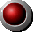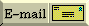Wind Turbines & Power Density

 How many windmills?   How much land area?     Compare with solar power density   Compare with serious power plant Wind turbines can't be spaced tip-to-tip, nor can they be closely lined up one behind the other.  Windmills slow down the air, and there's no point in putting windmills up where the air is guaranteed to be slow.  Therefore, wind turbines need to be spread out.  When you do so, how much power can you get out of how much land area? "Contemporary wind projects are typically rated at 25 to 100 MW.  A 25 MW project might have 60 to 70 turbines covering 1500 acres," says  The EPA .  Really, that's a little over 4 MW of *average* power from a total of about 65 windmills. (This was typical of early California wind turbines.) The 4 MW divided by 1500 acres is about 2.67 kW per acre.  But an acre is 4047 square meters, so the power density works out to be about 0.7 watts per square meter.  By comparison, direct sunlight averages 200 watts per square meter around the clock, around the year, around the US. Scale that up to 1000 MW (more or less standard for a serious power plant) by multiplying the number of windmills by 250.  That's over 16,000 windmills on about 375,000 acres (585 square miles). UPDATE 8/16/01  Murray County, WI. Navitas Energy, L.L.C. will erect 87 Enron 1.5-MW (nameplate) wind turbines on a 10,000 acre site.  This amounts to 13 kW (peak)/acre, or 3.2 watts per square meter of land area.  The wind turbines' diameter will be 70 meters.  Given an estimated 50% efficiency, the 1.5-MW output should be achieved at any speed over 10.7 meters per second (24 miles per hour), up to the wind spped where the windmill must be turned off. Assuming a  capacity factor  of 30%, the average power density will be 3.9 kW per acre (0.95 watts per square meter). Scale that up to 1000 MW around-the-clock power and the land area occupied would be 256,000 acres, which is 400 square miles. If the capacity factor were 40%, then the 1000-MW goal could be met with only 300 square miles of wind turbines.
To main Energy Advocate IndexYour comments & questions, please